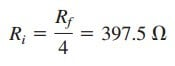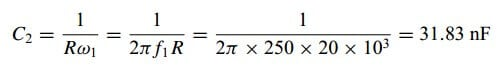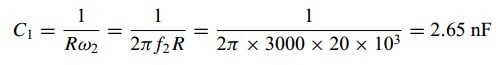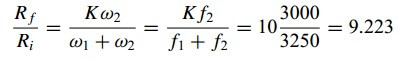# Simple Explanation of Active Filters TypesThe active filters have some advantages over the passive filters. There are three major limits to the passive filters considered in the previous post.

Contents

First, they cannot generate gain greater than 1; passive elements cannot add energy to the network. Second, they may require bulky and expensive inductors. Third, they perform poorly at frequencies below the audio frequency range (300 Hz < f < 3000 Hz).

Nevertheless, passive filters are useful at high frequencies.

## Active Filters

Active filters consist of combinations of resistors, capacitors, and op-amps. They offer some advantages over passive RLC filters.

First, they are often smaller and less expensive, because they do not require inductors. This makes feasible the integrated circuit realizations of filters.

Second, they can provide amplifier gain in addition to providing the same frequency response as RLC filters.

Third, active filters can be combined with buffer amplifiers (voltage followers) to isolate each stage of the filter from source and load impedance effects. This isolation allows designing the stages independently and then cascading them to realize the desired transfer function. (Bode plots, being logarithmic, may be added when transfer functions are cascaded.)

However, active filters are less reliable and less stable. The practical limit of most active filters is about 100 kHz—most active filters operate well below that frequency.

Filters are often classified according to their order (or the number of poles) or their specific design type.

### Active First-Order Lowpass Filter

One type of the first-order filter is shown in Figure.(1). The components selected for Zi and Zf determine whether the filter is lowpass or highpass, but one of the components must be reactive.

Figure.(2) shows a typical active low-pass filter.

For this filter, the transfer function is

where Zi = Ri and

Therefore,

We notice that Equation.(3) is similar to equation in passive lowpass filter, except that there is a low frequency (ω 0) gain or dc gain of –Rf /Ri. Also, the corner frequency is

which does not depend on Ri. This means that several inputs with different Ri could be summed if required, and the corner frequency would remain the same for each input

### Active First-Order Highpass Filter

Figure.(3) shows a typical highpass filter.

As before,

where Zi = Ri + 1/jωCi and Zf = Rf so that

This is similar to equation in passive highpass filter, except that at very high frequencies →∞), the gain tends to Rf/Ri. The corner frequency is

### Active Bandpass Filter

The circuit in Figure.(2) may be combined with that in Figure.(3) to form a bandpass filter that will have a gain K over the required range of frequencies.

By cascading a unity-gain lowpass filter, a unity-gain highpass filter, and an inverter with gain Rf/Ri, as shown in the block diagram of Figure.(4a), we can construct a bandpass filter whose frequency response is that in Figure.(4b).

The actual construction of the bandpass filter is shown in Figure.(5).

The analysis of the bandpass filter is relatively simple. Its transfer function is obtained by multiplying Equations.(3) and (6) with the gain of the inverter; that is

The lowpass section sets the upper corner frequency as

while the highpass section sets the lower corner frequency as

With these values of ω1 and ω2, the center frequency, bandwidth, and quality factor are found as follows:

To find the passband gain K, we write Equation.(8) in the standard form of Bode Plots,

At the center frequency ω0 = √(ω1ω2), the magnitude of the transfer function is

We set this equal to the gain of the inverting amplifier, as

from which the gain K can be determined.

### Active Bandreject (or Notch) Filter

A bandreject filter may be constructed by parallel combination of a lowpass filter and a highpass filter and a summing amplifier, as shown in the block diagram of Figure.(6a).

The circuit is designed such that the lower cutoff frequency ω1 is set by the lowpass filter while the upper cutoff frequency ω2 is set by the highpass filter.

The gap between ω1 and ω2 is the bandwidth of the filter. As shown in Figure.(6b), the filter passes frequencies below ω1 and above ω2. The block diagram in Figure.(6a) is actually constructed as shown in Figure.(7).

The transfer function is

The formulas for calculating the values of ω1, ω2, the center frequency, bandwidth, and quality factor are the same as in Equations.(9) to (13).

To determine the passband gain K of the filter, we can write Equation.(17) in terms of the upper and lower corner frequencies as

Comparing this with the standard form in Bode Plots indicates that in the two passbands (ω 0 and ω → ∞) the gain is

We can also find the gain at the center frequency by finding the magnitude of the transfer function at ω0 = √(ω1ω2), writing

Again, the filters treated in this section are only typical. There are many other active filters that are more complex.

## Active Filters Examples

Let’s review the active filters examples below:

### Active Filters Example 1

Design a low-pass active filter with a dc gain of 4 and a corner frequency of 500 Hz.

Solution:
From Equation.(4), we find

The dc gain is

We have two equations and three unknowns. If we select Cf = 0.2 µF, then

andWe use a 1.6-kΩ resistor for Rf and a 400-Ω resistor for Ri. Figure.(2) shows the filter.

### Active Filters Example 2

Design a bandpass filter in the form of Figure.(5) to pass frequencies between 250 Hz and 3000 Hz and with K = 10. Select R = 20 kΩ.

Solution:
Since ω1 = 1/RC2, we obtainSimilarly, since ω2 = 1/RC1,From Equation.(16),If we select Ri = 10 kΩ, then Rf = 9.223Ri 92 kΩ.